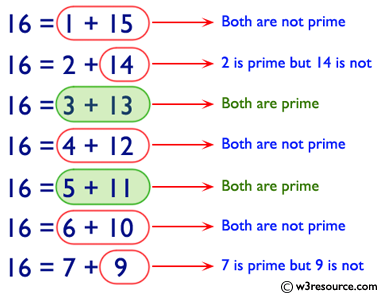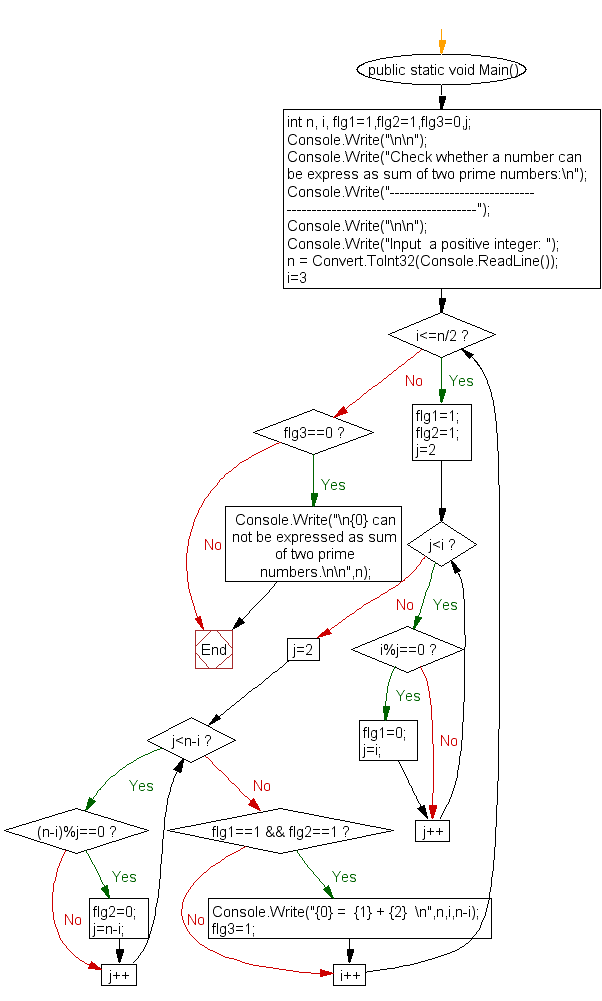﻿ C# - Check if a number can be express as sum of two prime# C# Sharp Exercises: Check whether a number can be express as sum of two prime numbers

## C# Sharp For Loop: Exercise-56 with Solution

Write a program in C# Sharp to check whether a number can be express as sum of two prime numbers.Sample Solution:-

C# Sharp Code:

``````using System;
public class Exercise56
{
public static void Main()
{
int n, i, flg1=1,flg2=1,flg3=0,j;

Console.Write("\n\n");
Console.Write("Check whether a number can be express as sum of two prime numbers:\n");
Console.Write("-------------------------------------------------------------------");
Console.Write("\n\n");

Console.Write("Input  a positive integer: ");
for(i=3; i<=n/2; i++)
{
/*---------- check for prime---------------*/
flg1=1;
flg2=1;
for(j=2; j<i; j++)
{
if(i%j==0)
{ flg1=0;j=i;}
}
for(j=2; j<n-i; j++)
{
if((n-i)%j==0)
{ flg2=0;j=n-i;}
}
if(flg1==1 && flg2==1)
{ Console.Write("{0} =  {1} + {2}  \n",n,i,n-i);
flg3=1;}
}
if(flg3==0)
{Console.Write("\n{0} can not be expressed as sum of two prime numbers.\n\n",n);}
}
}
```
```

Sample Output:

```Check whether a number can be express as sum of two prime numbers:
-------------------------------------------------------------------
Input  a positive integer: 50
50 =  3 + 47
50 =  7 + 43
50 =  13 + 37
50 =  19 + 31
```

Flowchart:C# Sharp Code Editor:

Contribute your code and comments through Disqus.

What is the difficulty level of this exercise?

Test your Programming skills with w3resource's quiz.

﻿